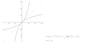# Natural parametrization of a curve

• A
• eva_92

#### eva_92

Hello,
I need the natural parametrization or a geodesic curve contained in the surface z=x^2+y^2, that goes through the origin, with x(s=0)=0, y(s=0)=0, dx/ds (s=0)=cos(a) and dy/ds(s=0)=sin(a), with "a" constant, expressed as a function of the arc length, i.e., I need r(s)=r(x(s),y(s)).
Thank you very much!
Eva

Hi. The surface is made by rotating the graph of ##z=x^2## around z axis. The shortest curve between the Origin and Point ##(X,Y,Z)## on the surface is presented by parameter ##\xi## as ##(X \xi,Y \xi,Z\xi^2)## where ##Z=X^2+Y^2,0<\xi<1##.

$$ds^2=X^2 d\xi^2 + Y^2 d\xi^2 + (X^2+Y^2)^2 4\xi^2 d\xi^2=(X^2 + Y^2)[ (X^2+Y^2)4\xi^2+1 ]d\xi^2$$
$$s(\xi)=\sqrt{X^2 + Y^2} \int_0^\xi \sqrt{ (X^2+Y^2)4\eta^2+1}\ d\eta$$
After calculating ##s(\xi)## you can get its inverse ##\xi(s)## and thus get ##(X\xi(s),Y\xi(s),Z\xi(s)^2)## as geodesic from Origin to end point (X,Y,Z) expressed by parameter s, the length from Origin.

Last edited:
Hello, thank you for the answer. I have tried with the parametrization x=rcos(a), y=rsin(a), z=r^2, reaching to a similar expression: ds^2=1+4*r^2 (similar to yours with X=cos(a), Y=sin(a)). The solution is s = (1/4)*ln⁡(2*r+sqrt(1+4*r^2))+(1/2)*r*sqrt(1+4*r^2), which is an equation in which I can not calculate r as a function of s to perform the natural parametrization. This is the reason of my question, maybe another parametrization could work, buy it does not happen to me. Could you help me with this?

You show points on geodesic
$$(r \cos a, r \sin a, r^2 )$$
where ##0<r<R## corresponding to the end point and
$$s = \frac{1}{4}ln⁡(2r+\sqrt{1+4r^2})+\frac{1}{2}r\sqrt{1+4r^2}$$
I would like to know what more you want.

Last edited:
The idea is to write x,y,x as a function of s, i.e., x(s),y(s),z(s). If I can calculate r(s), then I can write x(r(s)),y(r(s)),z(r(s)), but I can't calculate r(s) from that equation

I am afraid it is a tough thing to get the formula of r(s) from s(r) formula.

#### Attachments

•Screenshot_2020-12-20 inverse function of s = (1 4) ln⁡(2 r+sqrt(1+4 r^2))+(1 2) r sqrt(1+4 r^...png
4.2 KB · Views: 136